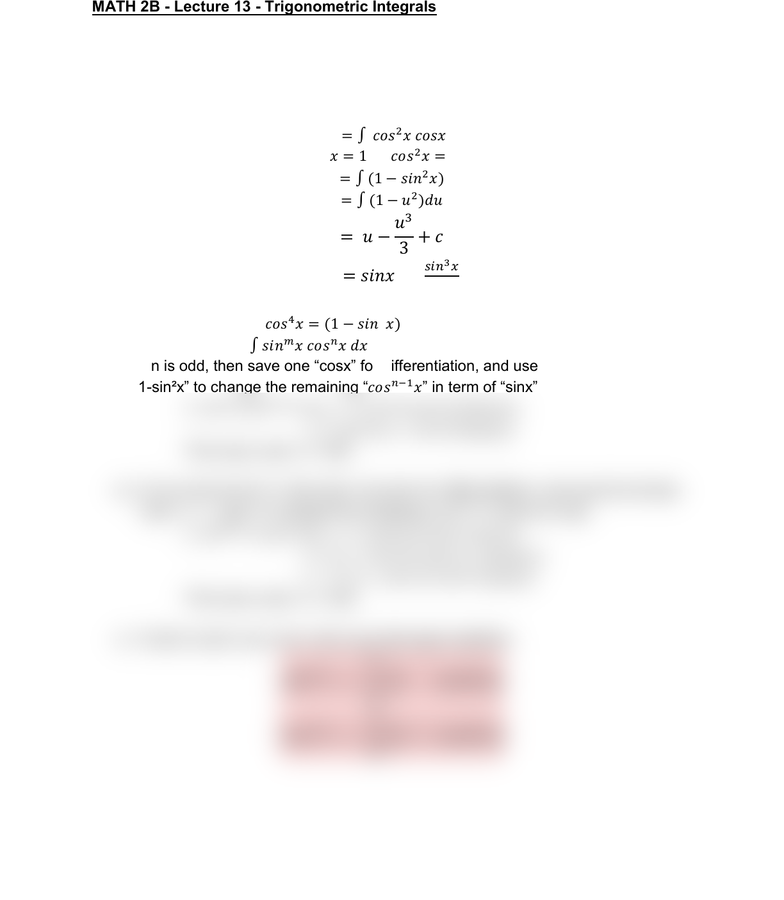Class Notes (1,100,000)
US (460,000)
UC-Irvine (10,000)
MATH (1,000)
MATH 2B (600)
Lecture 13

# MATH 2B Lecture 13: Trigonometric IntegralsPremium

Department
Mathematics
Course Code
MATH 2B
Professor
ERJAEE, G.
Lecture
13

This preview shows half of the first page. to view the full 2 pages of the document.02/04/2019
MATH 2B - Lecture 13 - Trigonometric Integrals
Example:
 
Solution:
    
Since       
 u=sinx


 
Because cos²x = 1-sin²x,  .
Strategy for evaluating .
a) If n is odd, then save one “cosxfor differentiation, and use the formula “cos²x =
1-sin²xto change the remaining in term of “sinx
 
 
Then use u-sub: u = sinx
b) If m is odd (m=2k+1), then save one sinx for differentiation, and use the formula
“sin²x = 1 - cos²xto change the remaining in term of cosx.
 
 
 
Then use u-sub: u = cosx
c) If both m and n are even, then use half-angle identities:



